Latest Banking jobs   »   Profit & Loss Basic Quantitative Aptitude...

# Profit & Loss Basic Quantitative Aptitude Quiz for All Banking Exams- 13th May

Q1. What will be the single discount equivalent to two successive discounts of 25% and 24%?
(a) 46%
(b) 43%
(c) 40%
(d) 33%
(e) 49%

Q2. I bought 16 pencils at the rate of Rs 9 per dozen and sold all of them at the rate of Rs 12 per dozen. What is the overall profit percentage in this transaction?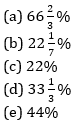Q3. A seller marks up an article 160% above of its cost price. After it he allows two successive discount of 20% and 25% and yet he gets 56% profit on it. If marked price of article is Rs 520 then find its selling price
(a) Rs 320
(b) Rs 312
(c) Rs 240
(d) Rs 324
(e) Rs 230

Q4. A milkman buys some milk. If he sells it at Rs 5 per litre, he loses Rs 300, but when he sells it at Rs 6 per litre, he gains Rs 250. How much milk did he purchase?
(a) 550 lt
(b) 300 lt
(c) 250 lt
(d) 800 lt
(e) 650 lt

Q5. A trader sells two articles for Rs. 4,800 each, neither losing nor gaining in total. If he sold one of the articles at a gain of 20%, the other is sold at a loss of what percent?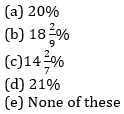Q6. How many kg of tea worth Rs. 25 per kg must be blended with 30 kg of tea worth Rs. 30 per kg so that by selling the blended variety at Rs. 30 per kg there should be gain of 10%?
(a) 30 kg
(b) 32 kg
(c) 36 kg
(d) 42 kg
(e) 34 kg

Q7. The price of a product after getting 10% discount is Rs.9450 which includes 5% tax on selling price. Find the marked price of the product (in Rs)?
(a) 8500
(b) 9000
(c) 10000
(d) 9500
(e) 10500

Q8. A shopkeeper in place of selling a article at 10% profit, mark up the article 30% above cost price and gives a discount of 10%. In this process he gets Rs. 56 more profit. Find the cost price of article.
(a) Rs. 400
(b) Rs. 1000
(c) Rs. 800
(d) Rs. 600
(e) Rs. 500

Q9. A person purchases four bicycles at a discount of 20% on marked price on each bicycle. If sum of discount offered and profit obtained on all 4 bicycles is 2560 then find the difference in marked price and cost price on one bicycle.
(a) 520
(b) 640
(c) 1220
(d) 880
(e) 690

Q10. Veer buy an article for Rs. 480. He sold it at 12% loss and get some money and from that money he again buys an article and this he sold at 25% profit. What was profit percentage he got from this transaction?
(a) 16%
(b) 12%
(c) 10%
(d) 14%
(e) 20%

Q11. A vegetable seller buy potato and tomato in Rs. 7.5 per kg. He sells potato at a profit of 22% and tomato at a loss of 8%, What is the S.P. of tomato, if in whole transaction there is no profit no loss ?
(a) 5.08 Rs.
(b) 4.97Rs.
(c) 5.07Rs.
(d) 5.06Rs.
(e) 5.66Rs.

Q12. In a basket there are some oranges. 33⅓% oranges were stolen by a thief, 30% of the remaining were rotten and rest oranges were good. If no. of good oranges was 140 then find total no. oranges in the basket.
(a) 300
(b) 350
(c) 320
(d) 400
(e) 420

Q13. A shopkeeper marks up the price of a T.V. 33⅓% above its cast price and gives a discount of 16⅔% on marked price. If cost price of the T.V. is Rs. 4500 then find the profit (in rupees)
(a) 1500
(b) 1000
(c) 500
(d) 400
(e) 800

Q14. A person sells a table at a profit of 10%. If he had bought the table at 5% less cost and sold for Rs. 80 more, he would have gained 20%. The cost price of the table is
(a) Rs. 3,200
(b) Rs. 2,500
(c) Rs. 2,000
(d) Rs. 3,000
(e) Rs. 1,600

Q15. Ritika bought a mobile at Rs. 18,000 after two successive discounts of 20% and 25%. Find the marked price of mobile.
(a) Rs. 30,000
(b) Rs. 25,000
(c) Rs. 36,000
(d) Rs. 40,000
(e) Rs. 24,000

Practice More Questions of Quantitative Aptitude for Competitive Exams:

Solutions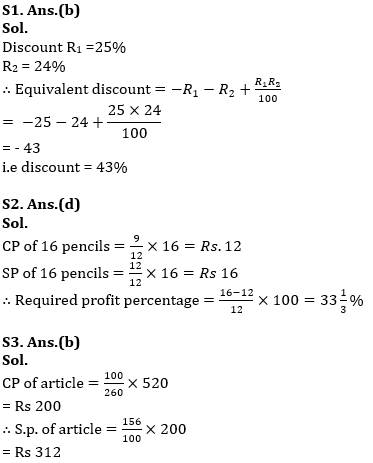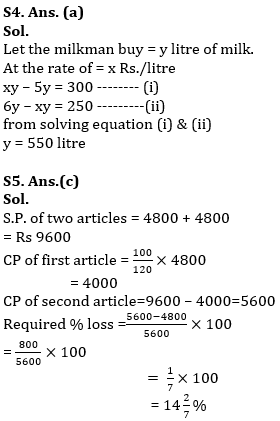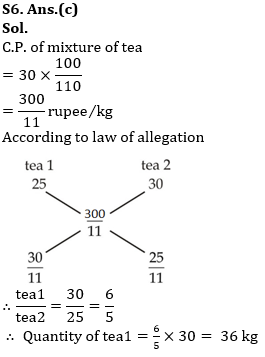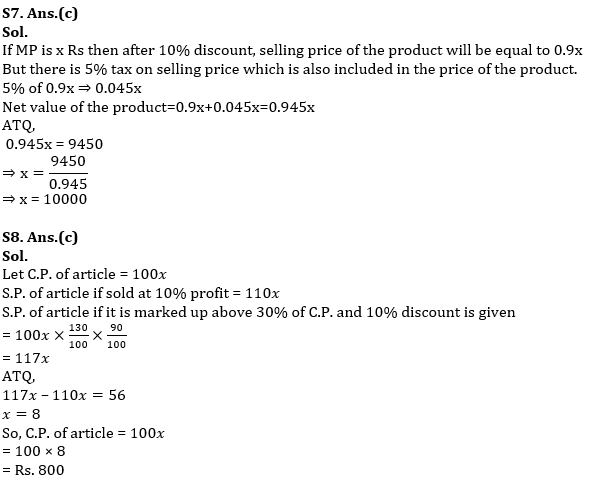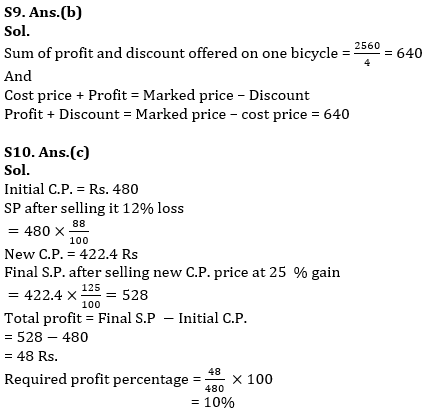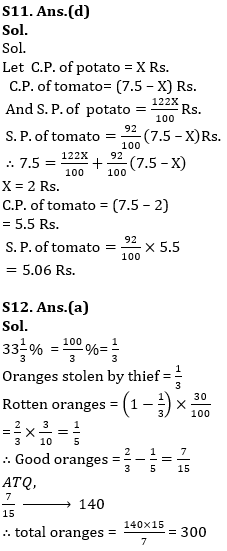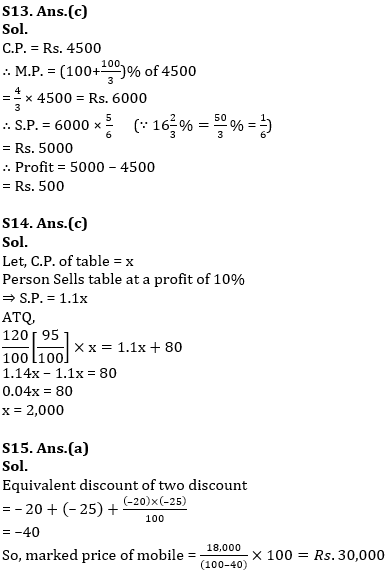#### Congratulations!Incorrect details? Fill the form again here

•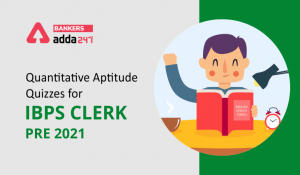Quantitative Aptitude Quiz For IBPS Cler...
•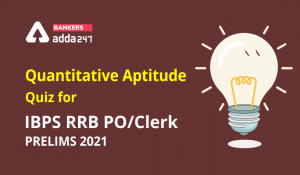Quantitative Aptitude Quiz For IBPS RRB ...
•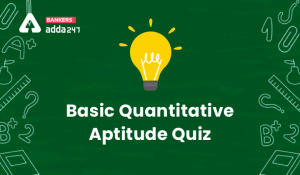Basic Quantitative Aptitude Quiz for All...
•Basic Quantitative Aptitude Quiz for All...
•Pie Chart DI Basic Quantitative Aptitude...
•Pie Chart DI Basic Quantitative Aptitude...